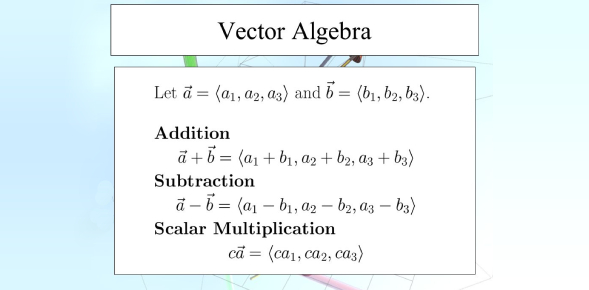Vector Algebra

15 Questions | Total Attempts: 582SettingsTime: 30 Minute

• 1.
• A.

4

• B.

5

• C.

6

• D.

7

• 2.
• A.

4

• B.

-4

• C.

5

• D.

6

• 3.
Write the position vector of the mid-point of the vector joining the points P (2, 3, 4) and Q (4, 1, — 2).
• A.
• B.
• C.
• D.
• 4.
• A.

0

• B.

1

• C.

2

• D.

3

• 5.
Write unit vector in the direction of the sum of vectors:
• A.
• B.
• C.
• D.
• 6.
P and Q are two points with position vectors and respectively. Write the position vector of a point R which divides the line segment PQ in the ratio 2:1 externally.
• A.
• B.
• C.
• D.
• 7.
• A.

0

• B.

1

• C.

3

• D.

4

• 8.
Using vectors, find the area of the triangle ABC with vertices A (1, 2, 3), B (2, -1, 4) and C (4, 5, -1).
• A.
• B.
• C.
• D.
• 9.
• A.

5

• B.

-5

• C.

Both A & B

• D.

7

• 10.
• A.
• B.
• C.
• D.
• 11.
Show that each of the given three vectors is a unit vector: Which of the following is true?
• A.
• B.
• C.
• D.

All of these

• 12.
• A.
• B.
• C.
• D.
• 13.
• A.
• B.
• C.
• D.
• 14.
• A.

-1

• B.

1

• C.

-1.5

• D.

2

• 15.
• A.

Points are collinear

• B.

Form a right angle triangle

• C.

Doesn’t form a right triangle

• D.

None of these

Related TopicsBack to top